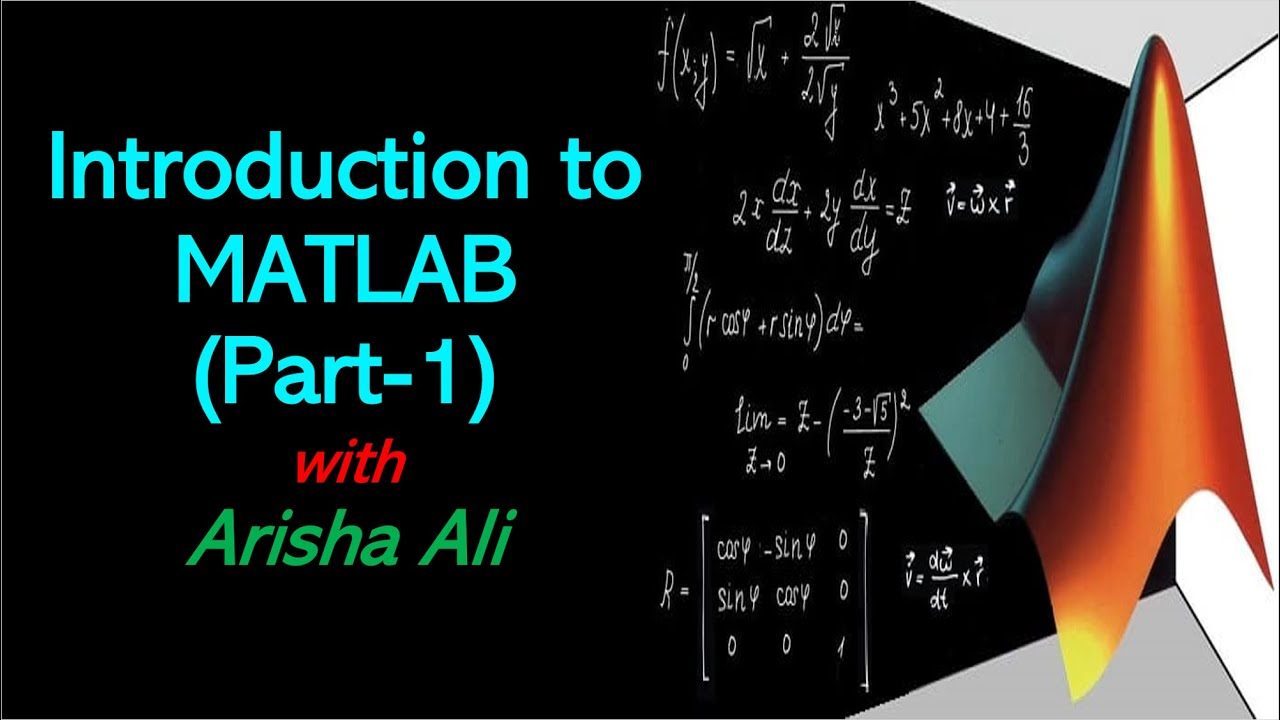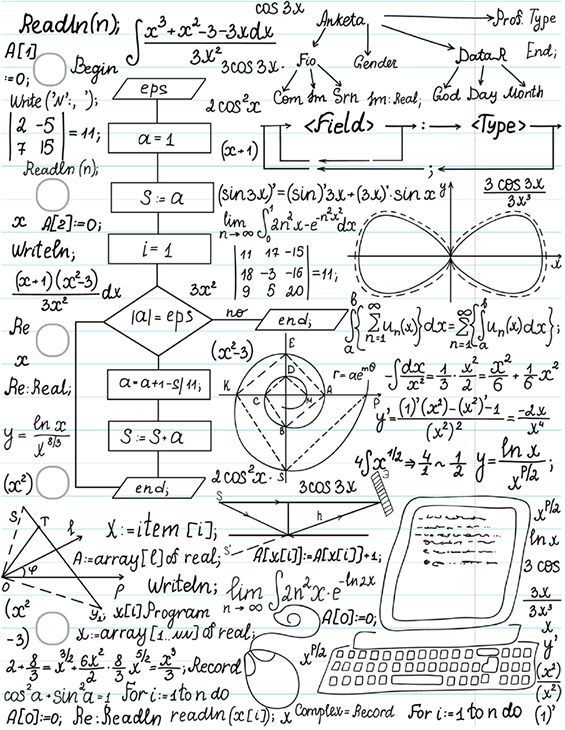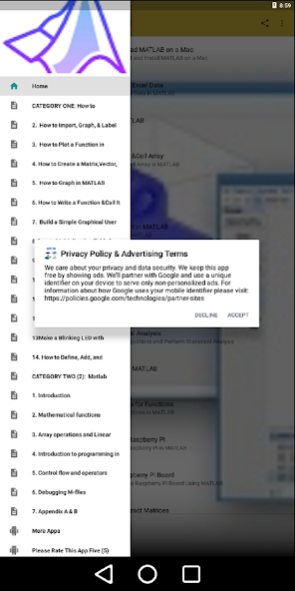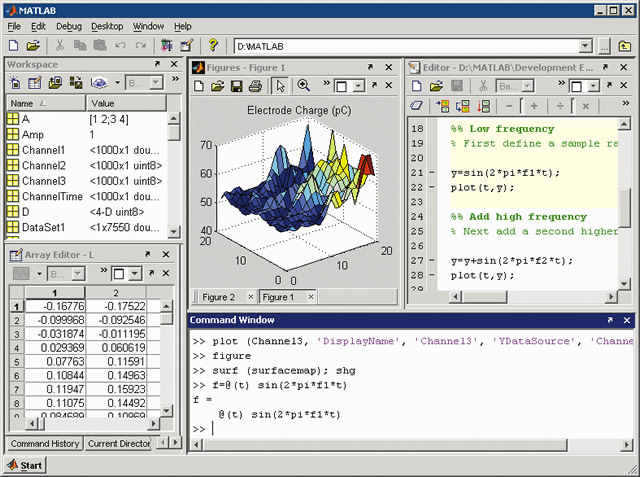# Matlab Presentation

Introduction to Matlab
Matlab is a high-level programming language and environment for numerical computation.

It is widely used in engineering, mathematics, and science fields.

Matlab provides a powerful set of tools for data analysis, visualization, and algorithm development.
Image Source1

Key Features of Matlab
Matlab has a large library of built-in functions and toolboxes for various applications.

It supports matrix operations and vectorized computations, making it efficient for handling large datasets.

Matlab has a user-friendly interface with a command window, editor, and debugger for easy coding and debugging.
Image Source2

Data Analysis and Visualization
Matlab provides a range of functions for data import, cleaning, and manipulation.

It offers powerful tools for statistical analysis, curve fitting, and modeling.

Matlab's visualization capabilities allow for creating 2D and 3D plots, animations, and interactive graphics.
Image Source3

Algorithm Development and Simulations
Matlab enables the development and implementation of complex algorithms and mathematical models.

It supports iterative and recursive programming techniques for algorithm optimization.

Matlab's simulation capabilities allow for testing and evaluating algorithms in various scenarios.
Image Source4

Integration and Deployment
Matlab can be integrated with other programming languages like C, C++, and Java.

It supports interoperability with external hardware devices and software systems.

Matlab code can be compiled into standalone executables or deployed as web applications.5

Resources and Community Support
Matlab has extensive documentation, tutorials, and examples for learning and reference.

The Matlab community offers online forums, user groups, and file exchange for sharing knowledge and code.

MathWorks, the company behind Matlab, provides technical support and training resources.
Image Source6

Conclusion
Matlab is a versatile and powerful tool for data analysis, algorithm development, and simulations.

It offers a user-friendly interface and a wide range of functions and toolboxes.

With its extensive resources and strong community support, Matlab is a go-to environment for scientific computing.
Image Source7